Back to index of science and math pages by Donald Sauter.

## The Area of Basic Geometric Figures

### ...with some little forays into mathematical thinking

*** AREA OF A RECTANGLE ***

Our starting point is the rectangle. It will be our jumping off point for the other basic 4-sided figures, as well as the triangle.

Suppose you have a rectangle 5 feet "long" and 3 feet "wide". Perhaps it's the floor inside a closet. Sorry about using the fuddy-duddy, old British unit, but my first loyalty is to my fellow Americans, and I think square feet are still a lot more comfortable and familiar than square decimeters, or square millimeters, or square hectometers, or whatever Europe buys floor tiles in. (Just kidding, but find my proposal for the simplest, most sensible system of units of measure.)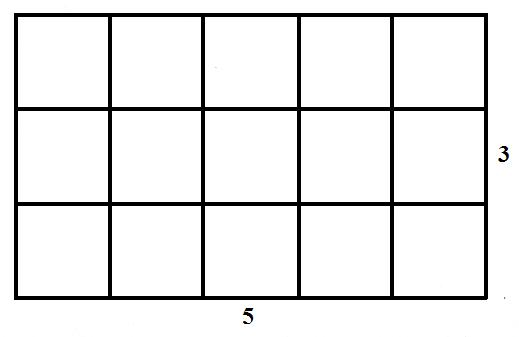Anyone can see that 3 rows of 5 tiles requires 15 square-foot tiles to cover the area. (If in doubt, count 'em up!) So, for the area of a rectangle, just multiply the length (L) times the width (W):

Area(rectangle) = L x W

What you hear in your head: "length times width".

You'll note the arbitrariness in specifying the order, L x W. Usually, in a picture, they'll call the long side the "length", and the shorter side the "width", and they'll have the figure sitting on the long side. (So much more stable that way!) But we don't care what letters they use -- could be A and B, or X and Y, or b and h, or anything -- or how the figure is oriented on the page; we know the area of a rectangle is simply the length of one of the sides times the length of the side right around the corner from it ("adjacent", you might say.)

To be honest, what my brain sees in this example is "3 rows of 5", that is, "3 times 5", as opposed to "5 times 3" as dictated by the formula. No matter; multiplication gives you the same answer same either direction. Which is good; imagine what a pain in the neck math would be otherwise!

An aside: Reversible Multiplication

Oops, I feel an aside coming on. But it's my web page, and not some stodgy, old text book. We all take for granted that multiplication gives the same answer both ways (is "commutative"), but if anyone asked you why, could you give an answer? For example, if this room has 17 tables with 13 place settings, and that room has 13 tables with 17 place settings, is it self-evident that both rooms have the same number of place settings?

I think the best answer is shown in our basic area example above. Three rows of 5 tiles (say) has to yield the same number of tiles as 5 rows of 3 tiles. It's the same picture in both cases! In our place setting example, imagine 17 neat rows of 13 "things". Then view the same layout as 13 rows of 17 things. I'll stick my neck out and theorize that this will work for any pair of numbers. :-) In any case, I've always found this kind of interesting -- even though "M sets of N things" certainly doesn't mean the same thing as "N sets of M things", good old multiplication comes up with the same number of things in each case.

Back to our Rectangle

Getting back to our specific example,

Area = L x W = 5 ft x 3 ft = 15 ft2

Or, 15 sq. ft. Don't worry, "square feet" and "feet squared" are two different, perfectly acceptable, ways of saying the exact same thing.

Another aside (already!)- Area with decimal and fractional lengths

I remember thinking as a kid, "Sure, that's as simple as pie for "natural" numbers like 1, 2, 3, etc., for lengths on each side, but what about something messy, like 5.7 feet by 3.2 feet? When we multiply 5.7 x 3.2 we get 18.24 square units. Can you visualize how the extra decimal bits will give the "just right" additional area outside the 5 x 3 rectangle, in this case, an extra 3.24 square units?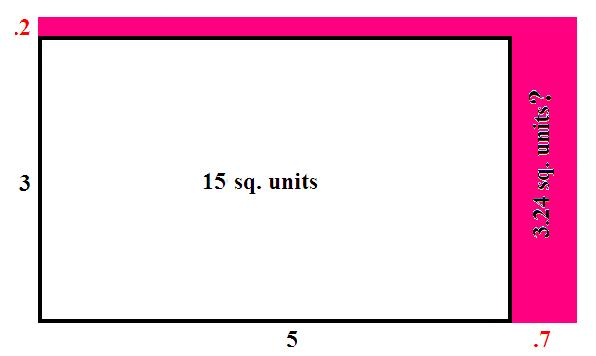If so, drop me a line. Sure, I can play with the math to tease out the extra 3.24, but I don't "see" it. But we're older and wiser, and don't even think about it. We know that numbers are numbers, and numbers are too trustworthy to have to worry about separate formulas for the different sorts of them. Just multiply L x W and be happy.

But if you're still a little worried, or just like flexing your math skills, you can put the problem back onto safe ground (natural numbers) by multiplying the lengths by 10 (in this case) to get rid of the decimals, and working in units which are 10 times smaller. In the original units, the area is 18.24; in the smaller units (where the unit of area is 10x10=100 times smaller) the area works out to 1824 complete, tiny tiles. Happy now? And you could do a similar thing with fractional lengths by working in units of the least common denominator.

Upward and onward...

*** AREA OF A SQUARE ***

Now, a square is just a special case of a rectangle, where all the sides are the same length. So the length (L) and the width (W) are the same, and we might as well just use one letter. A common choice is "s", for "side", so the area of a square is s times s, or "s squared".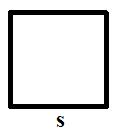Area(square) = s2

What you hear in your head: "ess squared", or, "the side squared".

We don't care what letter they use, "a" or "x" or whatever; just square it. (Hmmm... do you think this has anything to do with choosing the word "square" to mean multiplying a number by itself?)

*** AREA OF A TRIANGLE ***

I hope this resonates with you; it's really what gave me the nudge to work up this page in the first place. The area of a triangle is pretty simple, and a cinch to remember -- while you're doing lots of problems with it. But it's just hairy enough that most people will forget it after a period of disuse.

Does this sound familiar: "one half base times height"? Whether that's burned in, or sounds like greek, or anywhere in between, let's find a way to think about it so that we never have to trust it to our fading memories.

You can get the area of the triangle instantly from the area of our trusty rectangle -- and you'd have to lose every last marble to forget, "length times width." Take a rectangle, but instead of L and W for the sides, let's use "b" (for "base") and "h" (for "height"). After all, if you view the rectangle as standing up, the bottom side on the page is the base, and either upright side is the height.

So the area of our rectangle labeled in this way is A=bh. Now, slash a diagonal from one corner to the opposite corner and, voila!, you've made two triangles, each with an area obviously equal to half the area of the rectangle.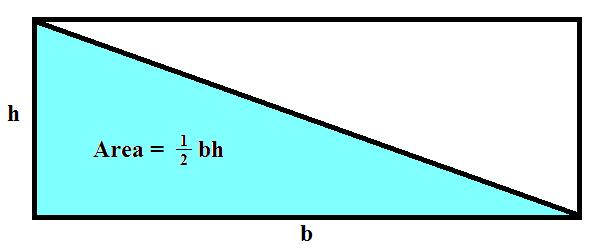Area(triangle) = 12 bh

What you hear in your head: "one half base times height".

Now, you may reasonably object, "But that's a special triangle! How do I know it will work for any triangle?"

Answer 1 is, if there are vestiges of "one half base times height" floating in you memory banks, this thought experiment should give you the "Oh, yeah, that's it!" feeling. We remember it wasn't a long, involved formula, surely no more complex than this, so this has to be it.

Answer 2 is, well, let's take a triangle that isn't special in any way, and play with that. Plop it on its longest side and call that the base (b). Snug up a rectangle around it. Drop a "height" (h) from the top point of the triangle. That splits our triangle into two smaller ones, and our rectangle into two smaller boxes. Look at each one separately.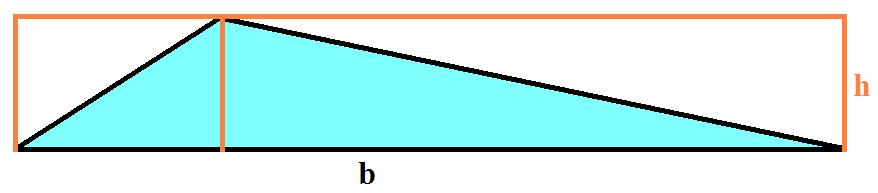One part of our triangle is half of the left box; the other part is half of the right box. Together, they're half of the whole box. Once again, and for sure this time,

Area(triangle) = 12 bh

*** AREA OF A PARALLELOGRAM ***

The parallelogram is a 4-sided figure (quadrilateral) in which the opposite sides have to be parallel. Imagine our trusty rectangle where somebody gave a firm, sideways push on the top to start to squash it down.What NOT to think: Oh, the parallelogram is just like a rectangle, so I'll just multiply b x c to get the area.

Wrong, wrong, wrong! But rather than try to see why that's wrong, let's take a look at what's right. Let's drop a height (h) from one of the corner points.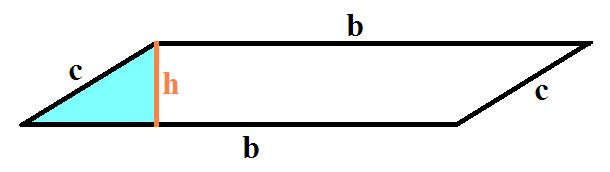That forms the triangle that I've shaded in. Now, let's slice off that triangle and drag it over to the other side, filling out a perfect rectangle. (We like rectangles!)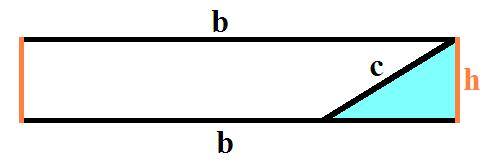And there you have the area of a parallelogram,

Area(parallelogram) = b x h

What you hear in your head: "base times height".

So you must know the height (h); just the lengths of the sides won't do it. Take a look back at our basic parallelogram. Do you see how if we pushed harder and harder on it, flattening it down almost completely, the area will keep getting smaller and smaller even though the lengths of the sides stay perfectly fixed?

*** Area of a Rhombus ***

Just as the square is a special case of a rectangle, with all sides equal, the rhombus is a special case of a parallelogram, with all sides equal.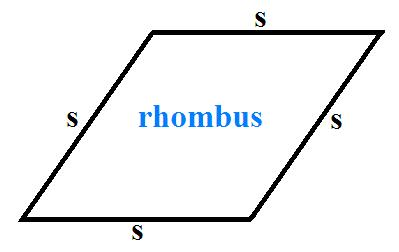The urge may be even stronger here to just multiply s x s to get the area, BUT DON'T DO IT! As I said, the rhombus is a parallelogram, so we have to know the height (h). Then we must multiply the base (which we're calling "s" here) times the height. Once again, drop a height from one of the corners, and visualize the triangle it forms dragged to the other side, forming a perfect rectangle (not a square!)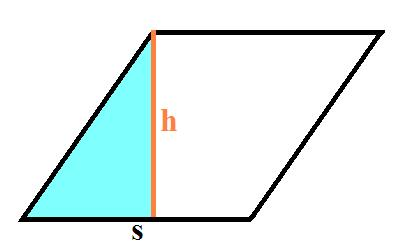Area(rhombus) = s x h

Even though we're using "s" for a side, what I hear in my head is still: "base times height". A rhombus is a parallelogram.

Now that we're totally comfortable with notion of "height" in our figures, think back on the rectangle and square. Notice those area formulas are really "base times height" as well - which had better be the case since they are parallelograms, too! It's just that for the rectangle and square, the right angles make the length of the sides "going up" the height. Not a big deal, but it's satisfying to see things in "the big picture".

*** AREA OF A TRAPEZOID ***

They define a trapezoid as a quadrilateral with two opposite sides parallel, and the other two sides NOT parallel. Understand that they did not consult me when they defined it. If they had, you can be sure a trapezoid would be any quadrilateral with a pair of parallel sides, with no constraint on the other pair. They might or might not be parallel. In which case we would have a very simple and logical hierarchy: There's the general quadrilateral; a trapezoid is a special case of the quadrilateral; a parallelogram is a special case of the trapezoid; a rectangle is a special case of the parallelogram; and a square is a special case of the rectangle. But noooooo... a parallelogram is not a trapezoid. (So they say...)

Here's a bare-bones, unlabeled trapezoid:Here it is again with some necessary labeling. Let's use big B for the length of the longer base; little b for the length of the shorter "base" (after all, we might've flipped the trapezoid onto its shorter parallel side); and h for the height.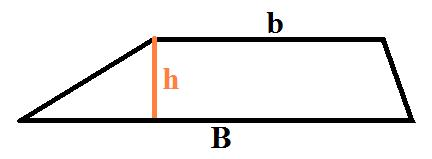Now, let's just blurt out the area of the trapezoid, and then think about how reasonable it is.

Area(trapezoid) = 12 (B + b) h

How do we remember that? Well, it looks a lot like the area of the triangle = 12 bh, but I recommend NOT thinking about the triangle. Think instead about our trusty rectangle. Clearly, using the big base, B, times the height would give too much area. Using the little base, b, would give too little area. But the AVERAGE of the two bases, 12 (B+b), is just right! (As Goldilocks would say...)

What you hear in your head: "the area of a trapezoid is the AVERAGE of the two bases times the height."

I will admit that, as reasonable as that may be, it's not obvious from the diagram. But I promise, with the most basic algebra you could prove the formula. If you want to, I'll set it up for you. View the trapezoid as consisting of three parts: a rectangle and two triangles.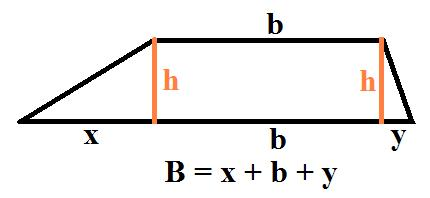Add up the areas of the rectangle and two triangles. Then, using the fact that x + b + y add up to B, work the total area into the form given above.

Quick aside: Did you notice that, when the little side, b, shrinks down to nothing (a point), the formula gives the area of a triangle (which is what it is!) Did you ever think about that? that a triangle is a special case of a trapezoid?

*** THE CIRCLE ***

The circle is a totally different beast from our 3- and 4-sided figures above, but while we're here let's run all of our basic geometric figures. We won't worry about deriving the area of the circle, just how to hang onto it for life.

You've heard it so many times, "pi r squared", that you're wondering, "Who could ever forget that?" The problem is that, whenever you see a circle in a math problem, you know one of two things is coming, either a question about the area, or a question about the circumference (the "perimeter" of the circle, or distance around its edge.)

And the two formulas are confusingly (and conveniently) similar. The area of the circle (as we said) is,

Area(circle) = π r2

And the circumference of a circle is, C = 2 π r

Note the exact same characters, just rearranged. But we are going to utilize this confusing similarity to our benefit. Get the sound of the two formulas burned in, just like you would, "Twinkle, twinkle, little star", and "Three blind mice."

"Pie are squared"; "pie are squared"; "pie are squared"...

"Two pie are"; "two pie are"; "two pie are"...

Don't worry about meaning; get the sayings going in your mind. Then you're loaded for (circular) bear, so to speak.

If your problem asks about the area of the circle, it has to be the formula with the "squared" in it. That's because area always comes in square units: square inches, square yards, square miles, square centimeters, etc., etc. Area calculations always involve multiplying one length times another. Think about our basic rectangle, with Area = Length x Width. (I view the multiplication of lengths as one of them "pulling" or "smearing" the other out into two dimensions, or into a surface. If that makes no sense to you, just forget I said anything!)

If the problem asks about the circle's circumference, which is just the length (or distance) around the circle, then it's a job for the "two pie are" formula. Which makes perfect sense, since the formula is just something multiplying a lone "r", and the radius is just a length.

Let me implore you NOT to think in terms of the Circumference = πD formula. Yes, that's correct. Yes, that's more concise by one symbol. But the conciseness simply does not make up for the confusion it introduces by juggling radius and diameter. It's the radius that "defines" the circle, anyhow; you swing a radius around a fixed point to make a circle. If someone gave you a string and asked for a circle with that as the diameter; you wouldn't know how to do it (until you got the idea to fold the string in half and swing that around a point.) And if you do start working with C = πD, you're sure to eventually goof up and either put the 2 back in (C = 2πD -- wrong!), or maybe even the factor that makes the area formula work with D (C = 14 πD -- wrong!) When the problem shows the diameter, just remember that the radius is half the diameter, and stick with the formulas using r. Thanks.

After all that, let me say it again,

Area(circle) = π r2

Circumference = 2 π r

What you hear in your head: both "pie are squared" and "two pie are", but choose the one you need based on the solo r (for simple length) or the r2 (for area).

There, we did it. And without a picture of a circle anywhere, or saying what "pi" is. I know you know!

Contact Donald Sauter: send an email; view guestbook; sign guestbook.
Back to Donald Sauter's main page.
Back to the top of this page.

